# NCERT Exemplar solutions for Class 11 Physics chapter 4 - Motion In a Plane [Latest edition]

#### Chapters## Solutions for Chapter 4: Motion In a Plane

Below listed, you can find solutions for Chapter 4 of CBSE NCERT Exemplar for Class 11 Physics.

Exercises
Exercises [Pages 19 - 28]

### NCERT Exemplar solutions for Class 11 Physics Chapter 4 Motion In a Plane Exercises [Pages 19 - 28]

#### MCQ I

Exercises | Q 4.1 | Page 19

The angle between A = hati + hatj and B = hati − hatj is ______.

• 45°

• 90°

• – 45°

• 180°

Exercises | Q 4.2 | Page 19

Which one of the following statements is true?

• A scalar quantity is the one that is conserved in a process.

• A scalar quantity is the one that can never take negative values.

• A scalar quantity is the one that does not vary from one point to another in space.

• A scalar quantity has the same value for observers with different orientation of the axes.

Exercises | Q 4.3 | Page 19

Figure shows the orientation of two vectors u and v in the XY plane.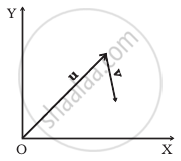If u = ahati + bhatj and v = phati + qhatj

which of the following is correct?

• a and p are positive while b and q are negative.

• a, p and b are positive while q is negative.

• a, q and b are positive while p is negative.

• a, b, p and q are all positive.

Exercises | Q 4.4 | Page 20

The component of a vector r along X-axis will have maximum value if ______.

• r is along positive Y-axis

• r is along positive X-axis

• r makes an angle of 45° with the X-axis

• r is along negative Y-axis

Exercises | Q 4.5 | Page 20

The horizontal range of a projectile fired at an angle of 15° is 50 m. If it is fired with the same speed at an angle of 45°, its range will be ______.

• 60 m

• 71 m

• 100 m

• 141 m

Exercises | Q 4.6 | Page 20

Consider the quantities, pressure, power, energy, impulse, gravitational potential, electrical charge, temperature, area. Out of these, the only vector quantities are ______.

• Impulse, pressure and area

• Impulse and area

• Area and gravitational potential

• Impulse and pressure

Exercises | Q 4.7 | Page 20

In a two dimensional motion, instantaneous speed v0 is a positive constant. Then which of the following are necessarily true?

• The average velocity is not zero at any time.

• Average acceleration must always vanish.

• Displacements in equal time intervals are equal.

• Equal path lengths are traversed in equal intervals.

Exercises | Q 4.8 | Page 20

In a two dimensional motion, instantaneous speed v0 is a positive constant. Then which of the following are necessarily true?

• The acceleration of the particle is zero.

• The acceleration of the particle is bounded.

• The acceleration of the particle is necessarily in the plane of motion.

• The particle must be undergoing a uniform circular motion.

Exercises | Q 4.9 | Page 21

Three vectors A, B and C add up to zero. Find which is false.

• (A × B) × C is not zero unless B, C are parallel

• (A × B) . C is not zero unless B, C are parallel

• If A, B, C define a plane, (A × B) × C is in that plane

• (A × B) . C = |A| |B| |C| → C2 = A2 + B2

Exercises | Q 4.10 | Page 21

It is found that |A + B| = |A|.This necessarily implies ______.

• B = 0

• A, B are antiparallel

• A, B are perpendicular

• A . B ≤ 0

#### MCQ II

Exercises | Q 4.11 | Page 21

Two particles are projected in air with speed vo at angles θ1 and θ2 (both acute) to the horizontal, respectively. If the height reached by the first particle is greater than that of the second, then tick the right choices

1. Angle of projection: q1 > q2
2. Time of flight: T1 > T2
3. Horizontal range: R1 > R2
4. Total energy: U1 > U2
Exercises | Q 4.12 | Page 21

A particle slides down a frictionless parabolic (y = x2) track (A – B – C) starting from rest at point A (Figure). Point B is at the vertex of parabola and point C is at a height less than that of point A. After C, the particle moves freely in air as a projectile. If the particle reaches highest point at P, then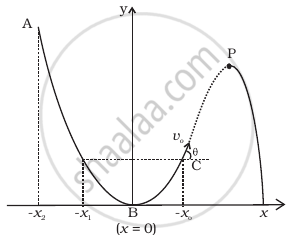• KE at P = KE at B

• height at P = height at A

• total energy at P = total energy at A

• time of travel from A to B = time of travel from B to P

Exercises | Q 4.13 | Page 21

Following are four differrent relations about displacement, velocity and acceleration for the motion of a particle in general. Choose the incorrect one (s):

1. v_(av) = 1/2 [v(t_1) + v(t_2)]
2. v_(av) = (r(t_2) - r(t_1))/(t_2 - t_1)
3. r = 1/2 (v(t_2) - v(t_1))(t_2 - t_1)
4. a_(av) = (v(t_2) - v(t_1))/(t_2 - t_1)
Exercises | Q 4.14 | Page 22

For a particle performing uniform circular motion, choose the correct statement(s) from the following:

1. Magnitude of particle velocity (speed) remains constant.
2. Particle velocity remains directed perpendicular to radius vector.
3. Direction of acceleration keeps changing as particle moves.
4. Angular momentum is constant in magnitude but direction keeps changing.
Exercises | Q 4.15 | Page 22

For two vectors A and B, A + B = A − B is always true when

1. |A| = |B| ≠ 0
2. A ⊥ B
3. |A| = |B| ≠ 0 and A and B are parallel or anti parallel
4. When either |A| or |B| is zero

#### VSA

Exercises | Q 4.16 | Page 22

A cyclist starts from centre O of a circular park of radius 1 km and moves along the path OPRQO as shown figure. If he maintains constant speed of 10 ms–1, what is his acceleration at point R in magnitude and direction?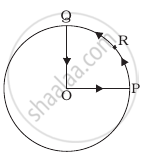Exercises | Q 4.17 | Page 22

A particle is projected in air at some angle to the horizontal, moves along parabola as shown in figure, where x and y indicate horizontal and vertical directions, respectively. Show in the diagram, direction of velocity and acceleration at points A, B and C.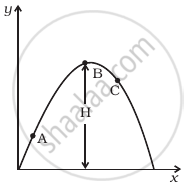Exercises | Q 4.18 | Page 23

A ball is thrown from a roof top at an angle of 45° above the horizontal. It hits the ground a few seconds later. At what point during its motion, does the ball have

1. greatest speed.
2. smallest speed.
3. greatest acceleration?

Explain

Exercises | Q 4.19 (a) | Page 23

A football is kicked into the air vertically upwards. What is its acceleration?

Exercises | Q 4.19 (b) | Page 23

A football is kicked into the air vertically upwards. What is its velocity at the highest point?

Exercises | Q 4.20 | Page 23

A, B and C are three non-collinear, non co-planar vectors. What can you say about direction of A × (B × C)?

#### SA

Exercises | Q 4.21 | Page 23

A boy travelling in an open car moving on a levelled road with constant speed tosses a ball vertically up in the air and catches it back. Sketch the motion of the ball as observed by a boy standing on the footpath. Give explanation to support your diagram.

Exercises | Q 4.22 | Page 23

A boy throws a ball in air at 60° to the horizontal along a road with a speed of 10 m/s (36 km/h). Another boy sitting in a passing by car observes the ball. Sketch the motion of the ball as observed by the boy in the car, if car has a speed of (18 km/h). Give explanation to support your diagram.

Exercises | Q 4.23 | Page 23

In dealing with motion of projectile in air, we ignore effect of air resistance on motion. This gives trajectory as a parabola as you have studied. What would the trajectory look like if air resistance is included? Sketch such a trajectory and explain why you have drawn it that way.

Exercises | Q 4.24 | Page 23

A fighter plane is flying horizontally at an altitude of 1.5 km with speed 720 km/h. At what angle of sight (w.r.t. horizontal) when the target is seen, should the pilot drop the bomb in order to attack the target?

Exercises | Q 4.25 (a) | Page 23

Earth can be thought of as a sphere of radius 6400 km. Any object (or a person) is performing circular motion around the axis of earth due to earth’s rotation (period 1 day). What is acceleration of object on the surface of the earth (at equator) towards its centre? what is it at latitude θ? How does these accelerations compare with g = 9.8 m/s2?

Exercises | Q 4.25 (b) | Page 23

Earth also moves in circular orbit around sun once every year with on orbital radius of 1.5 × 1011 m. What is the acceleration of earth (or any object on the surface of the earth) towards the centre of the sun? How does this acceleration compare with g = 9.8 m/s2?

Exercises | Q 4.26 | Page 24

Given below in column I are the relations between vectors a, b and c and in column II are the orientations of a, b and c in the XY plane. Match the relation in column I to correct orientations in column II.

 Column I Column II (a) a + b = c (i)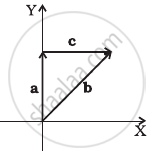(b) a – c = b (ii)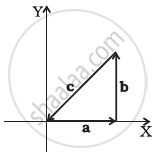(c) b – a = c (iii)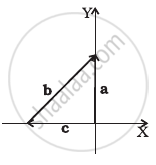(d) a + b + c = 0 (iv)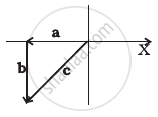Exercises | Q 4.27 | Page 25

If |A| = 2 and |B| = 4, then match the relations in column I with the angle θ between A and B in column II.

 Column I Column II (a) A.B = 0 (i) θ = 0 (b) A.B = + 8 (ii) θ = 90° (c) A.B = 4 (iii) θ = 180° (d) A.B = – 8 (iv) θ = 60°
Exercises | Q 4.28 | Page 25

If |A| = 2 and |B| = 4, then match the relations in column I with the angle θ between A and B in column II

 Column I Column II (a) |A × B| = 0 (i) θ = 30° (b) |A × B| = 8 (ii) θ = 45° (c) |A × B| = 4 (iii) θ = 90° (d) |A × B| = 4sqrt(2) (iv) θ = 0°

#### LA

Exercises | Q 4.29 | Page 25

A hill is 500 m high. Supplies are to be sent across the hill using a canon that can hurl packets at a speed of 125 m/s over the hill. The canon is located at a distance of 800 m from the foot of hill and can be moved on the ground at a speed of 2 m/s; so that its distance from the hill can be adjusted. What is the shortest time in which a packet can reach on the ground across the hill? Take g = 10 m/s2.

Exercises | Q 4.30 | Page 25

A gun can fire shells with maximum speed v0 and the maximum horizontal range that can be achieved is R = v_0^2/g. If a target farther away by distance ∆x (beyond R) has to be hit with the same gun (Figure), show that it could be achieved by raising the gun to a height at least h = Δx[ 1 + (Δx)/R]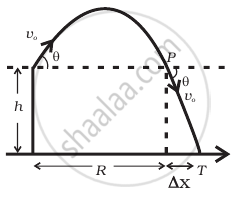Exercises | Q 4.31 | Page 26

A particle is projected in air at an angle β to a surface which itself is inclined at an angle α to the horizontal (Figure).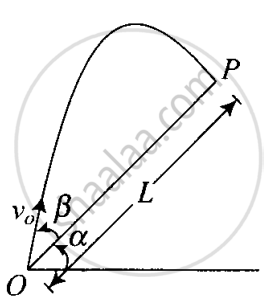1. Find an expression of range on the plane surface (distance on the plane from the point of projection at which particle will hit the surface).
2. Time of flight.
3. β at which range will be maximum.
Exercises | Q 4.32 | Page 26

A particle falling vertically from a height hits a plane surface inclined to horizontal at an angle θ with speed vo and rebounds elastically (Figure). Find the distance along the plane where if will hit second time.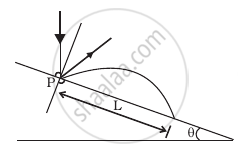Exercises | Q 4.33 | Page 27

A girl riding a bicycle with a speed of 5 m/s towards north direction, observes rain falling vertically down. If she increases her speed to 10 m/s, rain appears to meet her at 45° to the vertical. What is the speed of the rain? In what direction does rain fall as observed by a ground based observer?

Exercises | Q 4.34 | Page 27

A river is flowing due east with a speed 3 m/s. A swimmer can swim in still water at a speed of 4 m/s (Figure).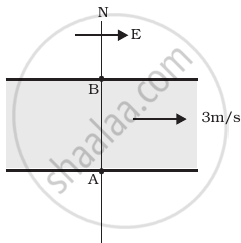1. If swimmer starts swimming due north, what will be his resultant velocity (magnitude and direction)?
2. If he wants to start from point A on south bank and reach opposite point B on north bank, (a) which direction should he swim? (b) what will be his resultant speed?
3. From two different cases as mentioned in (a) and (b) above, in which case will he reach opposite bank in shorter time?
Exercises | Q 4.35 | Page 27

A cricket fielder can throw the cricket ball with a speed vo. If he throws the ball while running with speed u at an angle θ to the horizontal, find

1. the effective angle to the horizontal at which the ball is projected in air as seen by a spectator.
2. what will be time of flight?
3. what is the distance (horizontal range) from the point of projection at which the ball will land?
4. find θ at which he should throw the ball that would maximise the horizontal range as found in (iii).
5. how does θ for maximum range change if u > vo, u = vo, u < vo?
6. how does θ in (v) compare with that for u = 0 (i.e.45)?
Exercises | Q 4.36 | Page 28

Motion in two dimensions, in a plane can be studied by expressing position, velocity and acceleration as vectors in cartesian co-ordinates A = A_xhati + A_yhatj where hati and hatj are unit vector along x and y directions, respectively and Ax and Ay are corresponding components of (Figure). Motion can also be studied by expressing vectors in circular polar co-ordinates as A = A_rhatr + A_θhatθ where hatr = r/r = cos θhati + sin θj and hatθ = - sin  θhati + cos θ hatj are unit vectors along direction in which r and θ are increasing.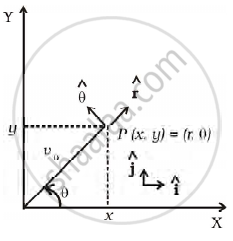1. Express hati and hatj in terms of hatr and hatθ
2. Show that both hatr and hatθ are unit vectors and are perpendicular to each other.
3. Show that d/(dt) (hatr) = ωhatθ where θ = (dθ)/(dt) and d/(dt) (hatθ) = - ωhatr
4. For a particle moving along a spiral given by t = aθhatr, where a = 1 (unit), find dimensions of ‘a’.
5. Find velocity and acceleration in polar vector representation for particle moving along spiral described in (d) above.
Exercises | Q 4.37 | Page 28

A man wants to reach from A to the opposite corner of the square C (Figure). The sides of the square are 100 m. A central square of 50 m × 50 m is filled with sand. Outside this square, he can walk at a speed 1 m/s. In the central square, he can walk only at a speed of v m/s (v < 1). What is smallest value of v for which he can reach faster via a straight path through the sand than any path in the square outside the sand?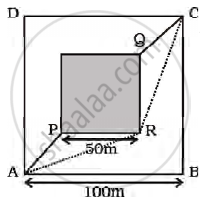## Solutions for Chapter 4: Motion In a Plane

Exercises## NCERT Exemplar solutions for Class 11 Physics chapter 4 - Motion In a Plane

Shaalaa.com has the CBSE Mathematics Class 11 Physics CBSE solutions in a manner that help students grasp basic concepts better and faster. The detailed, step-by-step solutions will help you understand the concepts better and clarify any confusion. NCERT Exemplar solutions for Mathematics Class 11 Physics CBSE 4 (Motion In a Plane) include all questions with answers and detailed explanations. This will clear students' doubts about questions and improve their application skills while preparing for board exams.

Further, we at Shaalaa.com provide such solutions so students can prepare for written exams. NCERT Exemplar textbook solutions can be a core help for self-study and provide excellent self-help guidance for students.

Concepts covered in Class 11 Physics chapter 4 Motion In a Plane are Motion in a Plane - Average Velocity and Instantaneous Velocity, Motion in a Plane - Average Acceleration and Instantaneous Acceleration, Angular Velocity, Scalar (Dot) and Vector (Cross) Product of Vectors, Relative Velocity in Two Dimensions, Cases of Uniform Velocity, Scalars and Vectors, Multiplication of Vectors by a Real Number or Scalar, Addition and Subtraction of Vectors - Graphical Method, Resolution of Vectors, Vector Addition – Analytical Method, Motion in a Plane, Motion in a Plane with Constant Acceleration, Projectile Motion, General Vectors and Their Notations, Rectangular Components, Uniform Circular Motion (UCM), Cases of Uniform Acceleration Projectile Motion, Introduction of Motion in One Dimension.

Using NCERT Exemplar Class 11 Physics solutions Motion In a Plane exercise by students is an easy way to prepare for the exams, as they involve solutions arranged chapter-wise and also page-wise. The questions involved in NCERT Exemplar Solutions are essential questions that can be asked in the final exam. Maximum CBSE Class 11 Physics students prefer NCERT Exemplar Textbook Solutions to score more in exams.

Get the free view of Chapter 4, Motion In a Plane Class 11 Physics additional questions for Mathematics Class 11 Physics CBSE, and you can use Shaalaa.com to keep it handy for your exam preparation.